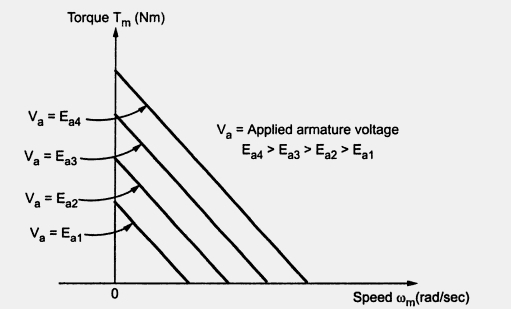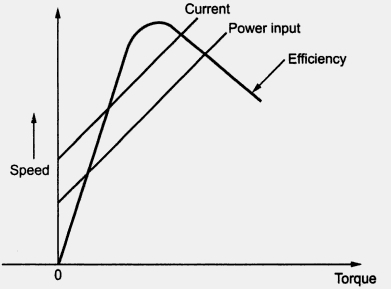### Characteristics of D.C. Servomotor

Servomotor Part 3-4
The torque-speed characteristics of a d.c. motor basically describes the static torque producing capability of the motor with respect to applied voltage and motor speed.
The effect of armature inductance is negligible in the steady state and hence the torque equation for a d.c. servomotor can be written as
where  Km  = Motor constant
Va  = Applied armature voltage
From the above equation (IV), it can be seen that the graph of Tagainst ωm   is a straight line which is dropping in nature, for constant value of Va . This line represents torque-speed characteristics of a d.c. servomotor, for constant Va
Fig. 3 shows a typical set of torque-speed characteristics for various values of applied voltage.Fig. 3 Torque-speed characteristics of d.c. servomotor

The slope of the characteristics can be obtained from the equation (IV), which is expressed as,

The slope of such characteristics is negative.
The torque-speed characteristics of d.c. servomotors are determined experimentally in practice, by conducting various tests on the servomotor.
1.1 Other Performance Characteristics
The performance characteristics other than the torque-speed characteristics of a d.c. servomotor are shown in the Fig. 4.Fig. 4 Performance characteristics of a typical d.c. servomotor

As torque developed increases, the current and power input increases while the efficiency increases upto certain limit and then starts decreasing.